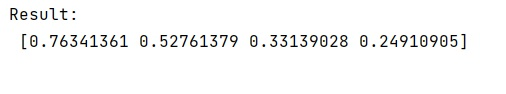# What does numpy.random.seed() do?

In this tutorial, we will learn about the numpy.random.seed() method, its usage and functionalities? Submitted by Pranit Sharma Last updated : May 23, 2023

## numpy.random.seed() Method

The random.seed() method is used to re-seed a Legacy BitGenerator. With the seed reset, the same set of numbers will appear every time, if the random seed is not reset, different numbers appear with every invocation.

Pseudo-random numbers work by standing with a number, multiplying it by a large number, adding an offset, then taking the modulo of that. The Sum of the resulting number is then used as the seed to generate the next random number. When you set the seed, it does the same thing every time giving you the same numbers.

### Syntax

```random.seed(seed=None)
```

### Parameter(s)

• seed: It is an optional parameter that is used to define seed for RandomState.

Let us understand with the help of an example,

## Example of numpy.random.seed() in Python

```# Import numpy
import numpy as np

# Using numpy random seed
res = np.random.rand(4)

# Display Result
print("Result:\n",res,"\n")
```

### Output